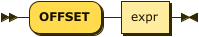SELECT Syntax

This page enables you to drill down through the syntax of a SELECT query.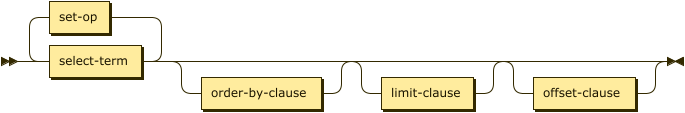select-term ::= subselect | '(' select ')'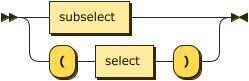subselect ::= select-from | from-select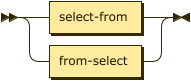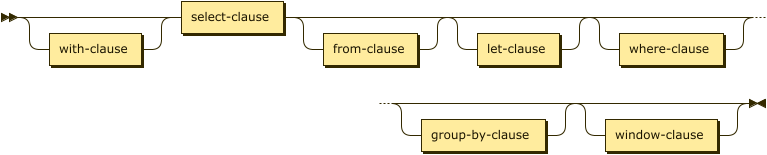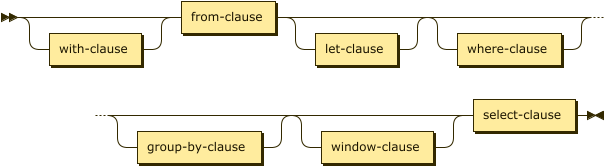set-op ::= UNION | INTERSECT | EXCEPT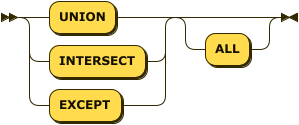SELECT Clause

select-clause ::= SELECT [ ALL | DISTINCT ] ( result-expr [ , result-expr ]* | ( RAW | ELEMENT | VALUE ) expr [ [ AS ] alias ] )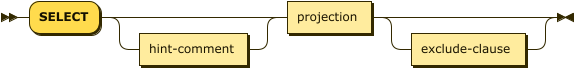FROM Clause

from-clause ::= FROM from-term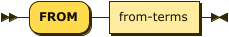from-keyspace ::= keyspace-ref [ [ AS ] alias ] [ use-clause ]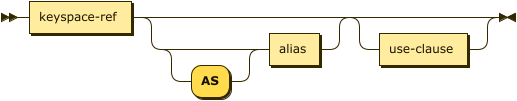keyspace-ref ::= [ namespace ':' ] keyspacenamespace ::= identifier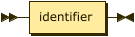keyspace ::= identifier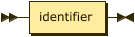from-subquery ::= subquery-expr [ AS ] aliassubquery-expr ::= '(' select ')'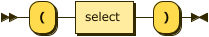from-generic ::= expr [ AS alias ]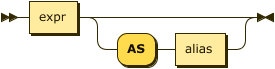JOIN Clause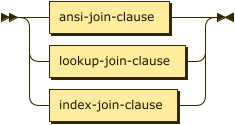ANSI JOIN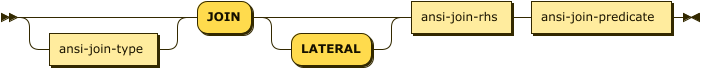ansi-join-type ::= INNER | ( LEFT [ OUTER ] ) | ( RIGHT [ OUTER ] )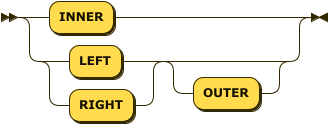ansi-join-rhs ::= keyspace-ref [ [ AS ] alias ]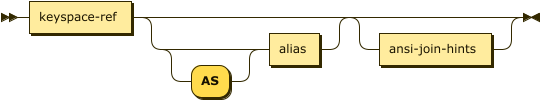ansi-join-hints ::= use-hash-hint | use-nl-hint | multiple-hintsuse-hash-hint ::= USE use-hash-term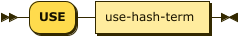use-hash-term ::= HASH '(' ( BUILD | PROBE ) ')'use-nl-hint ::= USE use-nl-term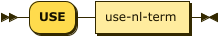use-nl-term ::= NL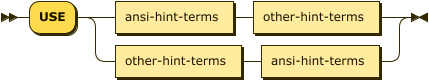ansi-hint-terms ::= use-hash-term | use-nl-termother-hint-terms ::= use-index-term | use-keys-termansi-join-predicate ::= ON expr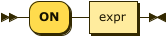Lookup JOIN

lookup-join-clause ::= [ lookup-join-type ] JOIN lookup-join-rhs lookup-join-predicate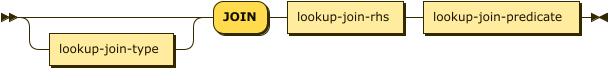lookup-join-type ::= INNER | ( LEFT [ OUTER ] )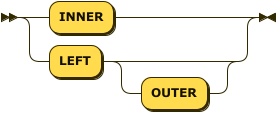lookup-join-rhs ::= keyspace-ref [ [ AS ] alias ]lookup-join-predicate ::= ON [ PRIMARY ] KEYS expr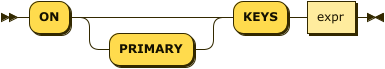Index JOIN

index-join-clause ::= [ index-join-type ] JOIN index-join-rhs index-join-predicate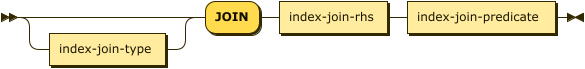index-join-type ::= INNER | ( LEFT [ OUTER ] )index-join-rhs ::= keyspace-ref [ [ AS ] alias ]index-join-predicate ::= ON [ PRIMARY ] KEY expr FOR alias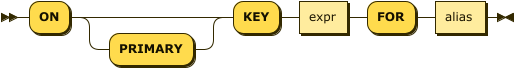NEST Clause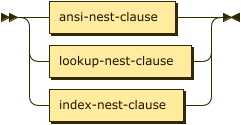ANSI NEST

ansi-nest-clause ::= [ ansi-nest-type ] NEST ansi-nest-rhs ansi-nest-predicate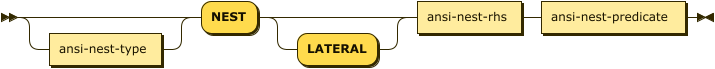ansi-nest-type ::= INNER | ( LEFT [ OUTER ] )ansi-nest-rhs ::= keyspace-ref [ [ AS ] alias ]ansi-nest-predicate ::= ON exprLookup NEST

lookup-nest-clause ::= [ lookup-nest-type ] NEST lookup-nest-rhs lookup-nest-predicate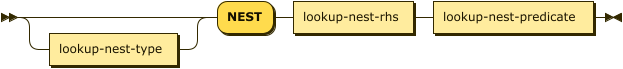lookup-nest-type ::= INNER | ( LEFT [ OUTER ] )lookup-nest-rhs ::= keyspace-ref [ [ AS ] alias ]lookup-nest-predicate ::= ON KEYS expr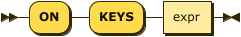Index NEST

index-nest-clause ::= [ index-nest-type ] NEST index-nest-rhs index-nest-predicate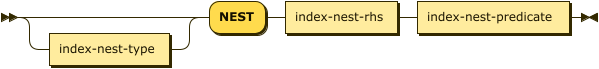index-nest-type ::= INNER | ( LEFT [ OUTER ] )index-nest-rhs ::= keyspace-ref [ [ AS ] alias ]index-nest-predicate ::= ON KEY expr FOR alias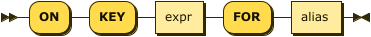UNNEST Clause

unnest-clause ::= from-term [ unnest-type ] ( UNNEST | FLATTEN ) expr [ [ AS ] alias ]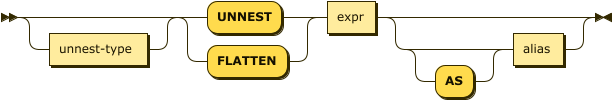unnest-type ::= INNER | ( LEFT [ OUTER ] )USE Clause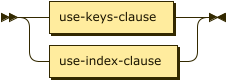use-keys-clause ::= USE use-keys-term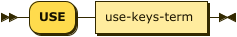use-keys-term ::= [ PRIMARY ] KEYS expruse-index-clause ::= USE use-index-term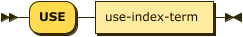use-index-term ::= INDEX '(' index-ref [ ',' index-ref ]* ')'index-ref ::= index-name [ index-using ]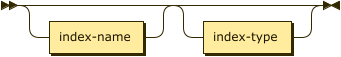index-name ::= identifierindex-using ::= USING ( VIEW | GSI )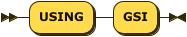LET Clause

let-clause ::= LET alias '=' expr [ ',' alias '=' expr ]*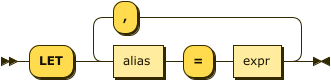WHERE Clause

where-clause ::= WHERE cond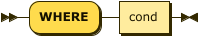cond ::= exprGROUP BY Clause

group-by-clause ::= GROUP BY expr [ ',' expr ]* [ letting-clause ] [ having-clause ] | letting-clause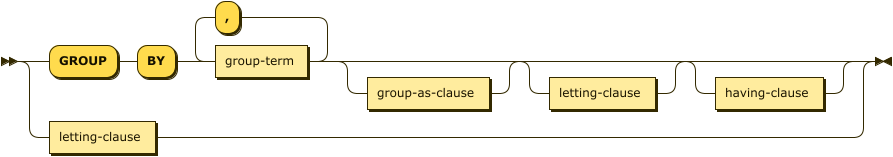letting-clause ::= LETTING alias '=' expr [ ',' alias '=' expr ]*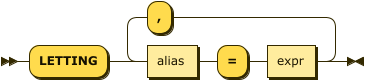having-clause ::= HAVING cond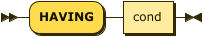ORDER BY Clause

order-by-clause ::= ORDER BY ordering-term [ ',' ordering-term ]*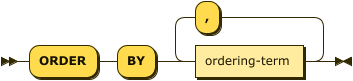ordering-term ::= expr [ ASC | DESC ]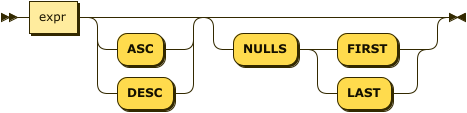LIMIT Clause

limit-clause ::= LIMIT expr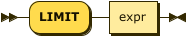OFFSET Clause

offset-clause ::= OFFSET expr